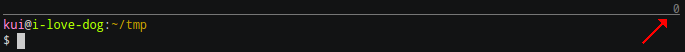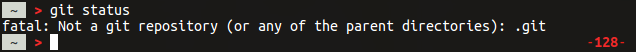Recently I came across this ASCII cast. My attention was caught by zsh configuration, that @kui is using. It prints exit code of last command before prompt:Let's look at the implementation.

https://github.com/kui/dotfiles/blob/master/dotfiles/zshrc#L411-L413

``````...
user="%F{\$(color_code_from_str "\$USER")}%n"
host="%F{\$(color_code_from_str "\$HOST")}%m"
PROMPT='%(?.%F{243}.%F{red})%U\${(l:COLUMNS:: :)?}%u
'"\$user"'%f@'"\$host"'%f:%F{yellow}%~ \$(for f in "\${prompt_opts[@]}"; "\$f")
%(?.%f.%F{red})%(!.#.\$)%f '
...
``````

Zsh has two prompts: default - `PROMPT` and the right prompt `RPROMPT`. The code above constructs default prompt using multiple pieces of data such as `\$USER` and `\$HOST` environment variables.

Let's look more closely at this:

``````%U\${(l:COLUMNS:: :)?}%u
``````
• `\$U` - anchor for starting underlined text.
• `(l:COLUMNS:: :)` - pads the left side of the parameter with spaces (given between the last two `: :`) until a width of \$COLUMNS is reached.
• `?` - in context of `\${...}` is equalent to `\$?` - a shell variable that store exit status code of last command.
• `%u` - the end of underlined text.

That's how it works. More about this can be found here:

I don't want to draw this line everytime, so I used a different approach:The exit code is displayed in right prompt only when it differs from zero (0 - a code for successful exit).

Here is the implementation from `.zshrc`:

``````...
function check_last_exit_code() {
local LAST_EXIT_CODE=\$?
if [[ \$LAST_EXIT_CODE -ne 0 ]]; then
local EXIT_CODE_PROMPT=' '
EXIT_CODE_PROMPT+="%{\$fg[red]%}-%{\$reset_color%}"
EXIT_CODE_PROMPT+="%{\$fg_bold[red]%}\$LAST_EXIT_CODE%{\$reset_color%}"
EXIT_CODE_PROMPT+="%{\$fg[red]%}-%{\$reset_color%}"
echo "\$EXIT_CODE_PROMPT"
fi
}
...
RPROMPT='\$(check_last_exit_code)\$(git_prompt_string)'
...
``````